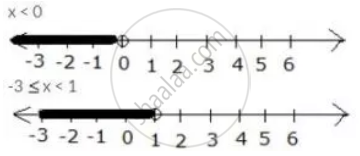Share

Use the Real Number Line to Find the Range of Values of X for Which: X < 0 and -3 ≤ X < 1 - ICSE Class 10 - Mathematics

ConceptRepresentation of Solution on the Number Line

Question

Use the real number line to find the range of values of x for which:

x < 0 and -3 ≤ x < 1

Solution

x < 0 and -3 ≤ x < 1

Both the given inequations are true in the range where their graphs on the real number lines overlap.

The graphs of the given inequations can be drawn as:From both graphs, it is clear that their common range is -3 ≤ x < 0

Is there an error in this question or solution?

APPEARS IN

Solution Use the Real Number Line to Find the Range of Values of X for Which: X < 0 and -3 ≤ X < 1 Concept: Representation of Solution on the Number Line.
S